# Assignement with teams of workers¶

## Problem description¶

In this example,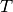tasks must be assigned to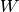workers. The workers are divided into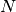teams and each team can have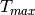tasks assigned at most. Also, a single worker cannot be assigned to more than one task. The cost of a task is a function of the assigned worker.

The aim is to minimize the total cost.

## Naive modelling¶

The problem can be exposed as follows:

Let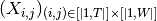be the set of binary variables indicating whether taskhas been assigned to worker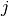. Let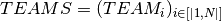with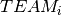the set of integers representing the workers of team. Let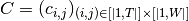with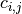the cost associated with the assignement of task. to worker.

The problem is then: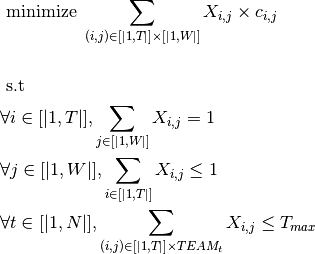## Implementation with standard API¶

The naive modelling could directly be implemented within Artelys Kalis solver. However, its large number of variables makes its resolution quite slow. By reducing this dimension, the following implementation improves drastically performance.

Previous modelling can be exposed as follows. Let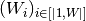be the set of variables taking its values in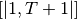indicating the task assigned to worker. Task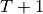is a dummy task, representing the “no-affectation”. Cost ofis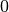. Letbe the set of teams withthe set of integers corresponding to the workers of team. Let matrix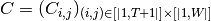be the cost associated to each task and worker.

The Problem is: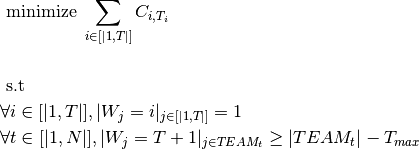The last constraint is the reciprocal of the naive modelling version : it asserts that enough workers in each team have been assigned to dummy task so not too many tasks are assigned to the team.

An implementation with Artelys Kalis is :

def solve(cost_matrix: list, teams_array: list, max_tasks_team: int):
""" Resolution of the 'team_of_worker' problem """

# Get the number of tasks and workers from cost_matrix and the number of teams from teams_array
nb_worker = len(cost_matrix)
nb_teams = len(teams_array)

try:

# Declaration of the problem
session = KSession()
problem = KProblem(session, "teams_of_worker")

# Variables declaration
workers_array = KIntVarArray()

# Loop over the workers to initialise the variables giving the task assigned to each one of them.
for worker in range(nb_worker):
workers_array += KIntVar(problem, "Worker_{}".format(worker), 0, nb_task)

# Assert that each task is assigned once

teams_tasks = {team: KIntVarArray() for team in range(nb_teams)}
# Loop over the teams to create a KIntVarArray for each of them in which are stored the tasks assigned to its
# workers
for team in range(nb_teams):
for worker in teams_array[team]:
# Assert that enough workers have the fictitious task for the team to not have too much tasks

# Objective declaration
# Transform the cost matrix into a Kalis object
K_cost_matrix = KIntMatrix(nb_worker, nb_task + 1, 0, "cost")
for worker in range(nb_worker):

# The objective will be greater than nb_task * the minimum of cost_matrix and lower than the sum of the
# coefficients in cost_matrix

# Transform the worker numbers into Kalis object to retrieve values from K_cost_matrix
num_worker = KIntVarArray()
for worker in range(nb_worker):
num_worker += KIntVar(problem, "Num_{}".format(worker), 0, nb_worker)
problem.post(num_worker[worker] == worker)

# Get the cost of the workers given the tasks they have been assigned
cost_worker = KIntVarArray()
for worker in range(nb_worker):
cost_worker += KIntVar(problem, "Cost_{}".format(worker), 0,
int(max([max(cost_matrix[worker]) for worker in range(nb_worker)])))
kelt = KEltTerm2D(K_cost_matrix, num_worker[worker], workers_array[worker])
problem.post(KElement2D(kelt, cost_worker[worker]))

# The objective is the sum of those costs
objective = KIntVar(problem, "cost", objective_inf, objective_sup)
problem.post(objective == sum(cost_worker[worker] for worker in range(nb_worker)))

# Set the objective
problem.setSense(KProblem.Minimize)
problem.setObjective(objective)

# Set the solver
solver = KSolver(problem)

# If the problem is infeasible
if problem.propagate():
# Display various details to help find the source of the problem
problem.display()
problem.printMinimalConflictSet()
print("Problem is infeasible")
sys.exit(1)

# Run optimization
result = solver.optimize()
if result:
solution = problem.getSolution()

# Display the solution
for worker in range(nb_worker):
print(f'Total cost : {solution.getValue(objective)}')

# Return the objective
problem.getSolution().display()
return solution.getValue(objective)

except ArtelysException as e:
print(e)

if __name__ == '__main__':
COST = [[90, 76, 75, 70],
[35, 85, 55, 65],
[125, 95, 90, 105],
[45, 110, 95, 115],
[60, 105, 80, 75],
[45, 65, 110, 95]]

TEAMS = [[0, 2, 4], [1, 3, 5]]

TEAM_MAX = 2

solve(COST, TEAMS, TEAM_MAX)


## Implementation with scheduling API¶

Even if the problem does not have a time dimension, it is possible to use the scheduling API to implement it. By considering a time period of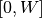, withthe number of workers, a single ressource with a capacity of 1, andtasks, the statment ‘task i start at j’ can be assimilated to the statment ‘task i has been assigned to worker j’.

A possible way to implement this method is as follows :

def team_of_workers(cost_matrix: list, teams_array: list, max_tasks_team: int):
""" Resolution of the 'team_of_worker' problem """

# Get the number of tasks and workers from cost_matrix and the number of teams from teams_array
nb_worker = len(cost_matrix)
nb_teams = len(teams_array)

try:

# Declaration of the problem
session = KSession()
problem = KProblem(session, "teams_of_worker")

# Creation of the schedule object with time horizon (0..nb_worker). The start date of a task will then be the
# number of the worker it is allocated to
schedule = KSchedule(problem, "schedule", 0, nb_worker)

# Setting up the resources which are the workers. They are discrete but can only take up to one task
workers = KDiscreteResource(schedule, "workers", 1)

# Setting up the tasks. Each task has a length of 1 - it requires only one worker - and consume one
# capacity of its worker

# Creation of an array linking a worker to its team
team_array = KIntArray()
for worker in range(nb_worker):
for team in range(nb_teams):
if worker in teams_array[team]:

# Creation of an array giving the teams of each worker having a task

# Get the costs of the tasks given the worker they have been assigned to
# Loop over the tasks to first get the workers it has been assigned to then store the team of the worker in

for team in range(nb_teams):

# The objective will be greater than nb_task * the minimum of cost_matrix and lower than the sum of the
# coefficients in cost_matrix

# Get the cost of the tasks given the workers they are assigned to
K_cost_worker, K_elt = {}, {}

0, max([cost_matrix[worker][task] for worker in range(nb_worker)]))

# The objective is the sum of those costs
objective = KIntVar(problem, "cost", objective_inf, objective_sup)

schedule.close()

# Set the objective
problem.setSense(KProblem.Minimize)
problem.setObjective(objective)

# Set the solver
solver = KSolver(problem)

# If the problem is infeasible
if problem.propagate():
# Display various details to help find the source of the problem
problem.display()
problem.printMinimalConflictSet()
print("Problem is infeasible")
sys.exit(1)

# Run optimization
result = solver.optimize()
if result:
solution = problem.getSolution()

# Display the solution
print('Total cost : ', solution.getValue(objective))

# Return the objective
problem.getSolution().display()
return solution.getValue(objective)

except ArtelysException as e:
print(e)

if __name__ == '__main__':
COST = [[90, 76, 75, 70],
[35, 85, 55, 65],
[125, 95, 90, 105],
[45, 110, 95, 115],
[60, 105, 80, 75],
[45, 65, 110, 95]]

TEAMS = [[0, 2, 4], [1, 3, 5]]

TEAM_MAX = 2

team_of_workers(COST, TEAMS, TEAM_MAX)